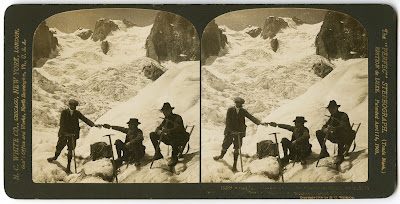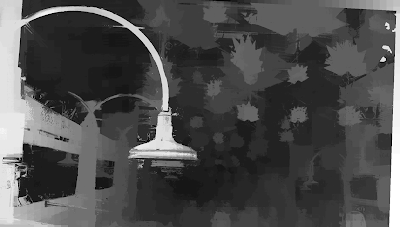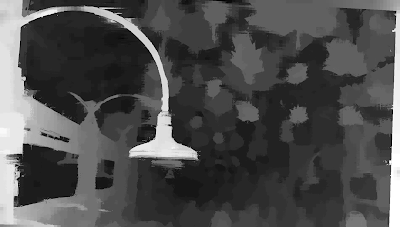## Saturday, December 2, 2017

### 3D Photos - Reversing Rapids in Saint-John, New Brunswick

Stereo pair taken with an HTC evo 3d phone. Size is 1887x1060 after auto-aligning in Stereo Photo Maker. I like to use the auto-alignment feature in Stereo Photo Maker and then click "Make an animation gif" to see if the wiggle/wobble that I intend to create via a depth map is gonna look good. Even though the stereo pair has been aligned in Stereo Photo Maker, I still rectify it with ER9b or ER9c.

Here is part of the output from ER9b:

Mean vertical disparity error = 0.457357
Min disp = -75 Max disp = 19
Min disp = -55 Max disp = 23

I am gonna use the rectified images to get a good depth map in a two-step process. ER9b gives me the min and max disparities in the 2nd of the 2 lines that give the min and max disparities (-55 and 23).

Step 1: Let's get a quick and dirty depth map using DMAG5b, a very rudimentary (but fast) depth map generator!

This is what I used for DMAG5b:

min disparity for image 1 = -55
max disparity for image 1 = 23
alpha = 0.9
truncation (color) = 30
disparity tolerance = 0
radius to smooth occlusions = 9
sigma_space = 9
sigma_color = 25.5

Because I am gonna use DMAG9b to improve the depth map quality, it's totally ok to use DMAG5b and it's totally ok to use it with a small radius like I did. Yes, there is quite a bit of noise but it really does not matter at this stage.

I am showing the corresponding occlusion map to give an idea of the quality of the depth map. Black means that the disparity in the depth map is probably not correct. Yes, there is quite a bit of black but no worries as DMAG9b will clean all that up nicely.

Step 2: Let's improve this noisy depth map with DMAG9b!

Parameters I used in DMAG9b:

sample_rate_spatial = 16
sample_rate_range = 8
lambda = 0.25
hash_table_size = 100000
nbr of iterations (linear solver) = 25
sigma_gm = 1
nbr of iterations (irls) = 32
gamma proximity (confidence map) = 12
gamma color similarity (confidence map) = 12
sigma (confidence map) = 32

I think this is a drastic improvement. DMAG9b is very good at improving depth maps, no matter where they come from.

The confidence map is computed by DMAG9b in the beginning of the computation. It shows the confidence in the disparities/depths in the input depth map (the one produced by DMAG5b) that DMAG9b has. The blacker the value, the less confidence DMAG9b will have in corresponding disparity/depth.

## Saturday, November 11, 2017

### 3D Photos - Old School Mountaineers

Let's see if we can get a depth map out of an old stereo card (provided by Paolo).This is an 1908 stereo card by H.C. White: A halt for refreshments on the Glacier du Géant, en route to Dent du Requin (in distance), Savoy, Alps.

First thing that needs to be done is to rectify the images, so that matches are always along the horizontal. We are gonna use ER9b to rectify the stereo pair.

Output from er9b (the important part):

Mean vertical disparity error = 0.384476
Min disp = -35 Max disp = 0
Min disp = -37 Max disp = 3

Here, we learn that the max vertical disparity error is rather small, which is very good. Ideally, it should be 0.0 (perfectly rectified images) but anything under 0.5 is excellent. As a bonus, we also get the min and max disparities: -37 and 3.

At this point, we kinda need to choose which depth map automatic generator to use. I tend to recommend using either DMAG5 or DMAG5b followed by DMAG9b. Let's try both combos!

List of all parameters used in DMAG5 (corresponding to depth map above):

alpha = 0.9
truncation (color) = 30
epsilon = 255^2*10^-4
disparity tolerance = 0
radius to smooth occlusions = 9
sigma_space = 9
sigma_color = 25.5
downsampling factor = 1

List of all parameters used in DMAG5 (corresponding to depth map above):

alpha = 0.9
truncation (color) = 30
epsilon = 255^2*10^-4
disparity tolerance = 0
radius to smooth occlusions = 9
sigma_space = 9
sigma_color = 25.5
downsampling factor = 1

List of all parameters used in DMAG5b (corresponding to depth map above):

alpha = 0.9
truncation (color) = 30
disparity tolerance = 0
radius to smooth occlusions = 9
sigma_space = 9
sigma_color = 25.5

List of all parameters used in DMAG5b (corresponding to depth map above):

alpha = 0.9
truncation (color) = 30
disparity tolerance = 0
radius to smooth occlusions = 9
sigma_space = 9
sigma_color = 25.5

Let's apply DMAG9b to all 4 depth maps and see what we get. We are gonna use the same DMAG9b parameters for all 4 depth maps.

List of all parameters used in DMAG9b (for all depth maps):

sample_rate_spatial = 32
sample_rate_range = 8
lambda = 0.25
hash_table_size = 100000
nbr of iterations (linear solver) = 25
sigma_gm = 1
nbr of iterations (irls) = 32
gamma proximity (confidence map) = 12
gamma color similarity (confidence map) = 12
sigma (confidence map) = 32

Not a whole lot of difference between all the depth maps after post-processing by DMAG9b. I am very tempted to say that it doesn't matter much whether you use DMAG5 or DMAG5b (or any of the other DMAG generators) to get the initial depth map (prior to applying DMAG9b). I tend to use the simplest depth map generator, that is, DMAG5b, to get the initial depth map. Note that one could play around with the DMAG9b parameters to attempt to improve the depth map quality, in particular, sample_rate_spatial (use 16 instead of 32 for example), sample_rate_range (use 16 or 4 instead of 8 for example), and lambda (use 2.5 or 0.025 instead of 0.25 for example).

## Thursday, September 28, 2017

### Case Study - DMAG5+DMAG9b

We are gonna use John Hooper's "People Waiting" in Saint-John, New Brunswick, to illustrate what DMAG9b can do to a depth map produced by DMAG5.

Sometime, ER9b is a bit too aggressive when rectifying and you end up with quite large camera rotations (resulting in a zooming effect). In those cases, I simply switch to ER9c, like in this case.

Let's get a "basic" depth map using DMAG5.

I used the following parameters in DMAG5:
alpha = 0.9
truncation (color) = 30
epsilon = 255^2*10^-4
disparity tolerance = 0
radius to smooth occlusions = 9
sigma_space = 9
sigma_color = 25.5
downsampling factor = 2

One could play around with the radius and epsilon to perhaps get a better depth map, but it's simpler to just let DMAG9b operate its magic.

I used the following parameters in DMAG9b:
sample_rate_spatial = 32
sample_rate_range = 8
lambda = 0.25
hash_table_size = 100000
nbr of iterations (linear solver) = 25
sigma_gm = 1
nbr of iterations (irls) = 32
gamma proximity (confidence map) = 12
gamma color similarity (confidence map) = 12
sigma (confidence map) = 32

Let's change the spatial sample rate from 32 to 16, leaving everything else as is, and let's rerun DMAG9b.

Let's change the spatial sample rate from 16 to 8, leaving everything else as is, and let's rerun DMAG9b.

The depth map looks pretty good, so we are gonna stop here.

### Case Study - DMAG5b+DMAG9b

The main parameter to tinker with when using DMAG5b is the radius. The lower the radius, the more noise. The larger the radius, the smoother the depth map is gonna be but the more inaccurate at object boundaries.

Let's stick with that depth map (for no particular reason) and post-process it with DMAG9b. The main parameters to tinker with in DMAG9b are the two sample rates (space and range aka color) and lambda (the smoothing multiplier).Depth map obtained by DMAG5b using radius = 16 and post-processed by DMAG9b using sample_rate_spatial = 32, sample_rate_range = 8, and lambda = 0.25.

What happens if you decrease the spatial sample rate from 32 to 16?Depth map obtained by DMAG5b using radius = 16 and post-processed by DMAG9b using sample_rate_spatial = 16, sample_rate_range = 8, and lambda = 0.25.

I think it's a bit better. I tried decreasing the range (color) sample rate from 8 to 4 but there was no improvement.

What happens if you increase lambda from 0.25 to 1.0?Depth map obtained by DMAG5b using radius = 16 and post-processed by DMAG9b using sample_rate_spatial = 16, sample_rate_range = 8, and lambda = 1.0.

Maybe it's a bit better although debatable. I tried to go the other way and decrease lambda but there was no improvement. So, let's call it a day and stop here.

## Wednesday, September 27, 2017

### 3D Image Conversion - Trinity

We are gonna use DMAG4 to semi-automatically convert a 2d image (still from "The Matrix" movie) into 3d via a depth map.

The "edge image" is simply a red trace (1 pixel wide) over the object boundaries. It takes about 5 minutes to do with the "Paths Tool". The "edge image" indicates depth changes and drastically simplifies the task of the dense depth map generator DMAG4. In the sparse depth map, I make use of the "Gradient Tool" when I need to establish depths along a slanted plane, here, along ground and gun. Otherwise, I just use the "Pencil Tool" ... very sparingly. I don't have to use the pencil much because of the presence of the "edge image".

I used the following parameters for DMAG4:
beta = 10
maxiter = 5000
scale_nbr = 1
con_level = 1
con_level2 = 1

Note the beta I am using. It's rather small. This makes DMAG4 kinda like an isotropic diffuser (of depths).

In wigglemaker, I chose "None" for inpainting method. I think it works best. Let me know if you don't think so! If you insist on relatively proper inpainting, it's probably a good idea to reduce the camera offset.

## Wednesday, September 6, 2017

### 3D Image Conversion - Top Gun

In this post, we are gonna look at 2d to 3d image conversion using DMAG4.

As you probably know, the input to DMAG4 is the 2d image, a sparse depth map, and possibly what I call an "edge image". The purpose of the edge image is to separate different objects in the scene. DMAG4 will not propagate depths past an edge in the edge image, which means you don't have to worry about the beta parameter.

I drew the "edge image" in Gimp using the "Paths" tool and stroking the path once done. Use the "Stroke Line" option, turn the anti-aliasing off, and choose 1 pixel for the width when stroking the path. It takes about 5 minutes to get the "edge image". Because I use an "edge image", DMAG4 can be allowed to propagate regardless of color similarity and therefore it is ok to use a small beta (makes the bilateral filter in DMAG4 behave like a regular Gaussian filter). Having an "edge image" makes things much easier when it is time to draw the sparse depth map and give the depth clues to DMAG4.

I took a pretty minimalist approach when drawing the sparse depth map, which means it is very sparse. To draw the sparse depth map in Gimp, I use the "Pencil" tool with an hard edge brush (no anti-aliasing). It takes another 5 minutes to draw the sparse depth map. When creating the sparse depth map, the important thing is the relationship between the various depths, not the actual depths. Make sure that when you zoom on the sparse depth map brushed on areas, the edges look jagged, which means there is no anti-aliasing applied, which is what we want.

We now have everything we need to launch DMAG4 and get the dense depth map.

I used the following parameters in DMAG4:
beta = 10
maxiter = 5000
scale_nbr = 1
con_level = 1
con_level2 = 1

Again, because I used an "edge image", I really don't have to worry about depths propagating across objects. This means that I can use a low beta (here, equal to 10). When beta is low, DMAG4 behaves pretty much like a classic Gaussian filter. If beta is large, DMAG4 behaves like a bilateral filter, in other words, it propagates depths only along similar colors, which can be a real problem in some cases. The idea behind using a bilateral filter is that things that are not of the same color probably don't belong to the same object and are probably at different depths. Of course, this is not ideal because you can clearly have different colors within an object. Because of that, if you don't use an "edge image", the sparse depth map may need to be not so sparse at all and a lot of time is wasted drawing the sparse depth map, running DMAG4, and fixing the sparse depth map before going through another iteration. Conclusion: use an "edge image" and a low beta!

## Thursday, August 24, 2017

### Case Study - DMAG5+DMAG9b vs DMAG5b+DMAG9b

In this post, I am gonna try to show that one can use DMAG5b instead of DMAG5 when the baseline is relatively small. I will also show the effects of selected parameters when using DMAG9b which is used to smooth (and sharpen) depth maps.

I took the stereo pair with an HTC Evo 3d cell phone which has a baseline of 35mm, I believe. The stereo pair is 1920x1080 pixels.

I used the following parameters for DMAG5:
min disparity for image 1 = -23
max disparity for image 1 = 27
disparity map for image 1 = depthmap_l.png
disparity map for image 2 = depthmap_r.png
occluded pixel map for image 1 = occmap_l.png
occluded pixel map for image 2 = occmap_r.png
alpha = 0.9
truncation (color) = 30
epsilon = 255^2*10^-4
disparity tolerance = 0
radius to smooth occlusions = 9
sigma_space = 9
sigma_color = 25.5
downsampling factor = 1

Since I am gonna use DMAG9b to smooth and sharpen the depth maps obtained by DMAG5b, it makes sense to also smooth and sharpen the depth map obtained by DMAG5 with DMAG9b.

I used the following parameters for DMAG9b:
sample_rate_spatial = 32
sample_rate_range = 8
lambda = 0.25
hash_table_size = 100000
nbr of iterations (linear solver) = 25
sigma_gm = 1
nbr of iterations (irls) = 32
gamma proximity (confidence map) = 12
gamma color similarity (confidence map) = 12
sigma (confidence map) = 32

Now that we have a point of reference, we can go ahead and see what happens when we use DMAG5b instead of DMAG5. As a reminder, DMAG5b is a very simplistic depth map generator that uses basic SAD (Sum of Absolute Differences) to find matches. This type of depth map generator always fatten object boundaries. The effect is more intense as the baseline increases and/or the radius increases.

I used the following parameters for DMAG5b:
min disparity for image 1 = -23
max disparity for image 1 = 27
disparity map for image 1 = depthmap_l.tiff
disparity map for image 2 = depthmap_r.tiff
occluded pixel map for image 1 = occmap_l.tiff
occluded pixel map for image 2 = occmap_r.tiff
alpha = 0.9
truncation (color) = 30
disparity tolerance = 0
radius to smooth occlusions = 9
sigma_space = 9
sigma_color = 25.5

The important parameter here is the radius. DMAG5b smoothes the disparities over a 2*radius+1 block window. To reduce the fattening of object boundaries, the only thing one can do is to reduce the radius but at the expense of increased noise.

I used the same parameters as before except:

I think it's tighter (than with radius = 16) so we are gonna use that depth map and sharpen it with DMAG9b.

I used the following parameters for DMAG9b:
sample_rate_spatial = 32
sample_rate_range = 8
lambda = 0.25
hash_table_size = 100000
nbr of iterations (linear solver) = 25
sigma_gm = 1
nbr of iterations (irls) = 32
gamma proximity (confidence map) = 12
gamma color similarity (confidence map) = 12
sigma (confidence map) = 32

Now, compare that depth map with the depth map we got with the combo DMAG5+DMAG9b and you will see that there's not a whole lot of difference between the two. So, I think it's fair to say that DMAG5b can safely be used in lieu DMAG5 as long as the obtained depth map is post-processed by DMAG9b.

Let's see what happens in the depth map produced by DMAG9b when we change the spatial sample rate and the range (color) sample rate.

I used the same parameters as before for DMAG9b except:
sample_rate_spatial = 32
sample_rate_range = 4

I used the same parameters as before for DMAG9b except:
sample_rate_spatial = 16
sample_rate_range = 8

I used the same parameters as before for DMAG9b except:
sample_rate_spatial = 16
sample_rate_range = 4

Now, it might be relatively fun to see what happens when we change the smoothing multiplier lambda. I am going back to sample_rate_spatial = 32 and sample_rate_range = 8 changing lambda only. We are gonna make the depth map less smooth first and then more smooth.

I used the following parameters for DMAG9b:
sample_rate_spatial = 32
sample_rate_range = 8
lambda = 0.025
hash_table_size = 100000
nbr of iterations (linear solver) = 25
sigma_gm = 1
nbr of iterations (irls) = 32